# Get Address of First Cell in Range

We will learn how to get address of first cell in range in this post. At Instance, we must determine the address of first cell in range to use as the data table’s value. For the DATA table, we have a range of value values in the form of an array that is sent to the formula as input, and therefore the formula returns the first cell value.

The next sections include information on the functions mentioned before that we will use.

ADDRESS retrieves the address of a cell using the row and column numbers.

## Syntax:

`=ADDRESS(row_num, col_num, [abs_num] )`
• `Row_num`: The Row number
• `Col_num`: The Column number
• `[abs num]`: [Optional] integer, one of the following values: 1, 2, 3, or 4. If this parameter is ignored or set to 1, the returned address will be absolute, e.g. \$A\$1.

Now we’ll combine these functions into a formula. We’ll begin with a data table. We need to determine the data table’s initial cell address.

Utilize the following formula:

`=ADDRESS(ROW(table_array), COLUMN(table_array))`

Variables:

• `table array`: array representation of a data table

## Examples:

Let us validate this formula by applying it to an example.

Here we have a data table and we need to use the formula to get the first cell address in the same data table.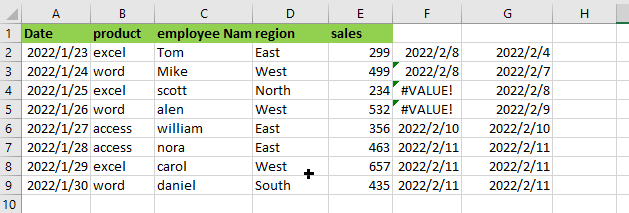Use the following Formula:

`= ADDRESS(ROW(A1:E9), COLUMN(A1:E9))`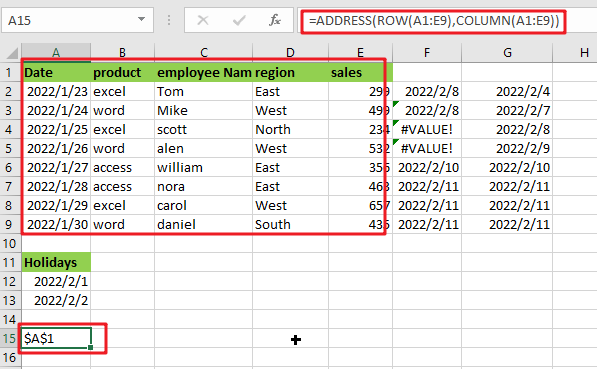Note: A1:E9 is array of tables

## Let’s See How This Formula Works:

`= COLUMN(A1:E9)`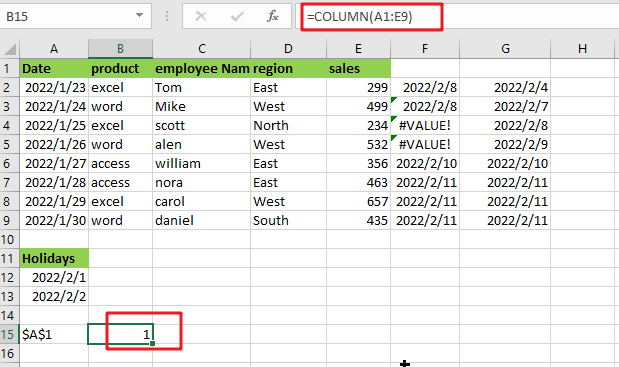The COLUMN function returns the value of the first column in the table array.

`= ROW(A1:E9)`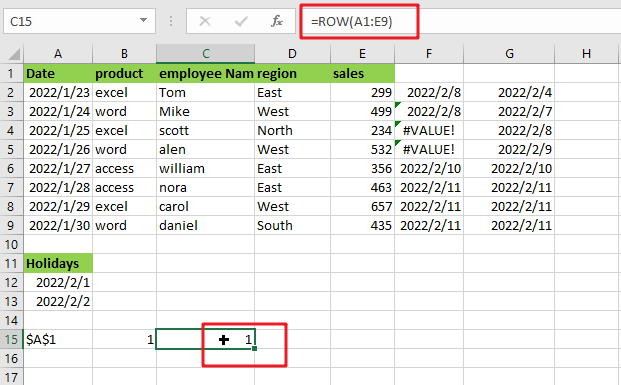The ROW function returns the index of the first row in the table array.

The ADDRESS function accepts the row number and column value parameters and returns the row’s and column’s absolute references.

The array sent to the function is denoted by cell reference. To get the result, press Enter.

As you can see, the formula works correctly since it delivers the value for the first cell in the data table.

Alternatively, you may use the CELL function:

CELL is an Excel built-in information function. Excel’s Cell function saves all the data in a specified cell and returns the cell’s info type. Using the follow formula:

`=CELL("address", [array_reference])`

or

`=CELL("address",INDEX(A1:E9,1,1))`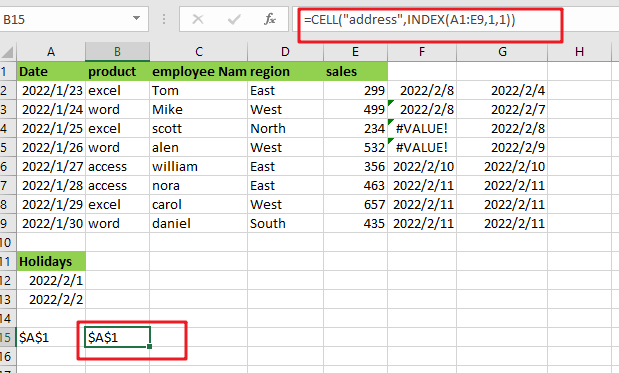Note:

• `[array_reference] `: table array given as reference.

As seen by the above calculation, you may use the first cell value as a reference.

The INDEX function can be used to get cell reference to the first cell in the given range by giving INDEX 1 for row number and 1 for column number.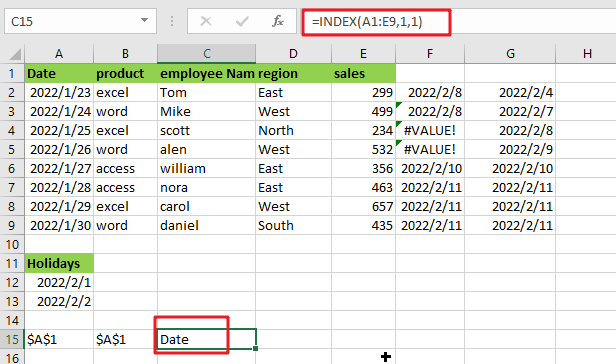The ADDRESS function may be used in conjunction with the ROW, COLUMN, and MIN functions to get the address of the first cell in a range. I hope this post on finding the get address of first cell in range in Excel was informative. It’s extremely easy and very helpful for people who use excel regularly.

### Related Functions

• Excel INDEX function
The Excel INDEX function returns a value from a table based on the index (row number and column number)The INDEX function is a build-in function in Microsoft Excel and it is categorized as a Lookup and Reference Function.The syntax of the INDEX function is as below:= INDEX (array, row_num,[column_num])…
• Excel ROW function
The Excel ROW function returns the row number of a cell reference.The ROW function is a build-in function in Microsoft Excel and it is categorized as a Lookup and Reference Function.The syntax of the ROW function is as below:= ROW ([reference])….
The Excel ADDRESS function returns a reference as a text string to a single cell.The syntax of the ADDRESS function is as below:=ADDRESS (row_num, column_num, [abs_num], [a1], [sheet_text])….
• Excel COLUMN function
The Excel COLUMN function returns the first column number of the given cell reference.The syntax of the COLUMN function is as below:=COLUMN ([reference])….
• Excel CELL function
The Excel CELL function returns information about the formatting, location, size, or contents of a cell.The syntax of the CELL function is as below:= CELL (info_type,[reference])…
• Excel MIN function
The Excel MIN function returns the smallest numeric value from the numbers that you provided. Or returns the smallest value in the array.The MIN function is a build-in function in Microsoft Excel and it is categorized as a Statistical Function.The syntax of the MIN function is as below:= MIN(num1,[num2,…numn])….
Related Posts

Creating a Table with Automatic Row Numbering

When creating an Excel Table, you may use a calculation relying on the ROW function to insert row numbers automatically. The formula in A2, which has been copied down, is as follows: =ROW()-ROW(Table1[#Headers]) Note: the default table name is Table1. ...

Abbreviate Names Or Words in Excel

As an MS Excel user, you might have come across a task where you need to abbreviate different names or words, and there are also possibilities that you might have done this task manually by assuming that there isn't any ...

Filter or Extract with a Partial Match

Assume you have a table consisting of a few cells having few values, and you want to filter out the set of records with the partial match. You might take it easy and would prefer to manually filter out the ...

Extract matching values From Two Lists

Suppose that you are working with two lists containing few values, and you want to extract the matching values from those two lists into another separate list. You might prefer to manually extract the matching values from the two lists, ...

Extract all Partial Matches

Just assume that you have a range of data that contains a text string and you want to extract the few partial matches into another separate range of cells; then you might think that it's not a big deal; because ...

Extract Multiple Match Values into Separate Columns

If you have a few values/items in the excel sheet and you are thinking that with the aid of the “VlOOKUP” function you can look for a specific value, extract it and then put the matching item into the separate ...

Average the Last N Numeric Values in Excel

AVERAGE function is one of the most popular functions in Excel. Apply AVERAGE together with some other functions, we can calculate average simply for some complex situations. In this article, we will introduce you to calculate average of the last ...

How To Sum the Largest N Values in Excel

Sometimes we may want to sum the largest N numbers or top N numbers in a range. In this article, we will show you the method of “SUM the Largest N Numbers” by a simple formula which consist of SUMPRODUCT ...

How to Sum the Smallest N Values in Excel

Sometimes we may want to sum the first smallest N numbers in a range in Excel. In this article, we will show you the method of “SUM the Smallest N Numbers” by a simple formula which consist of SUMPRODUCT and ...

How to Auto Fill Weekdays or Weekends in Excel

Sometimes we may want to enter a sequence of days in excel for some purpose, and we can press Ctrl+; to insert current date into cell, and then drag the cell down to attach following days into other cells, then ...

Sidebar